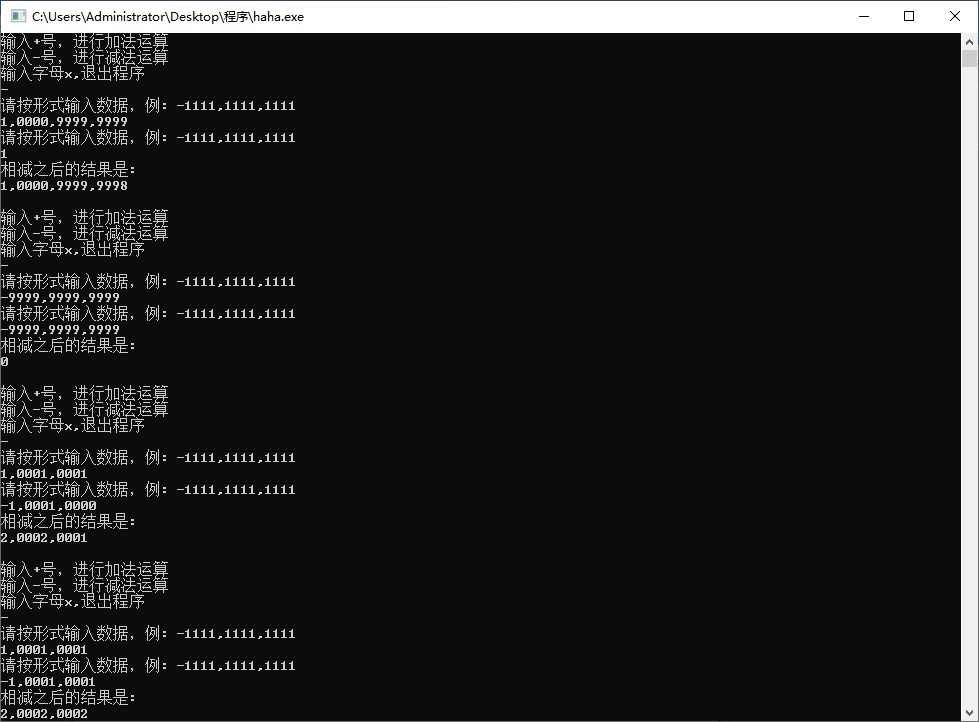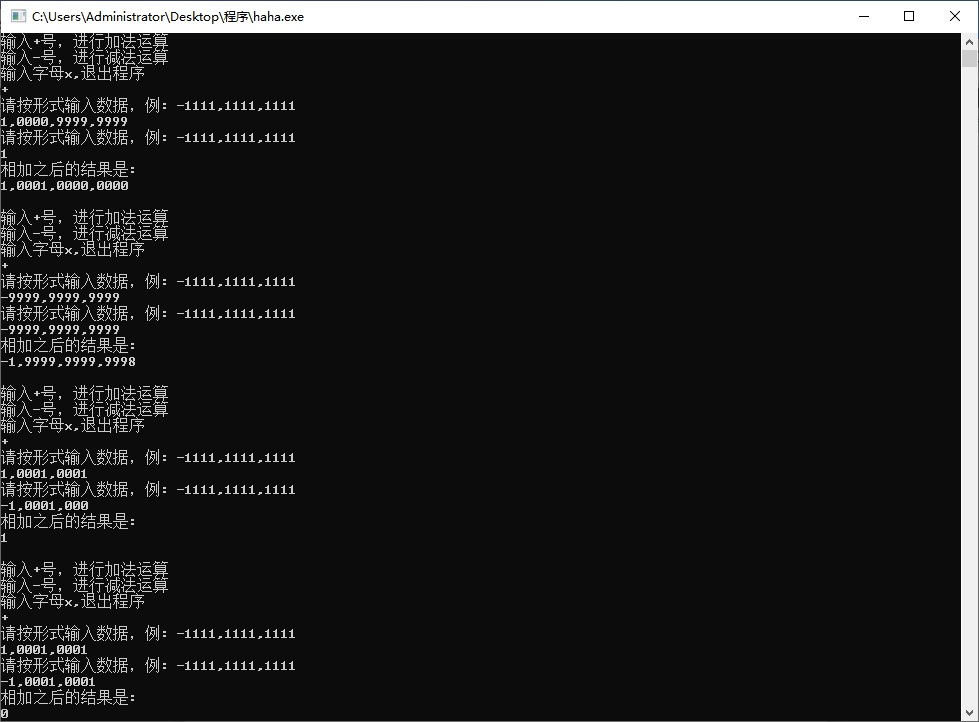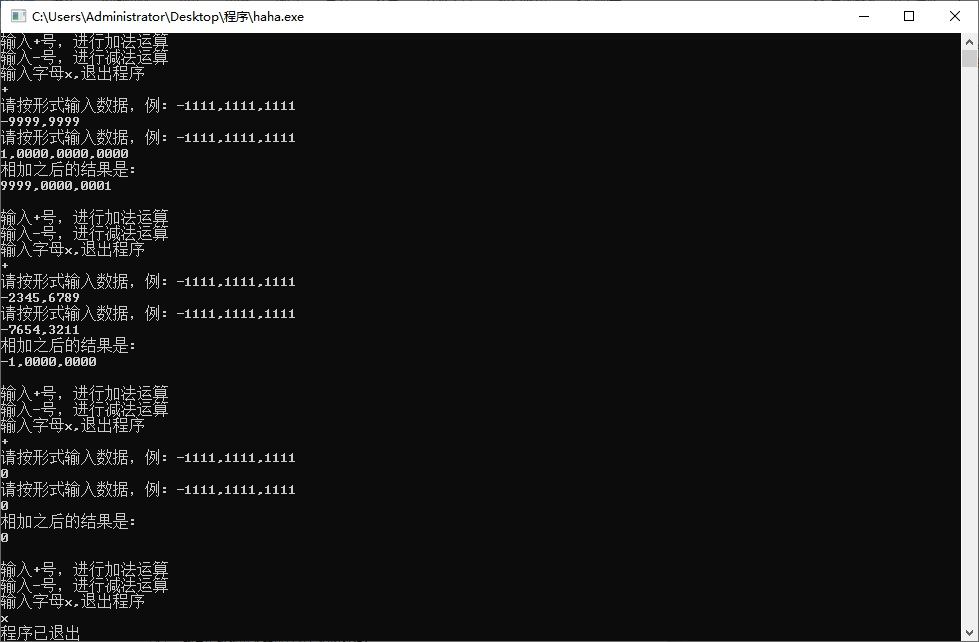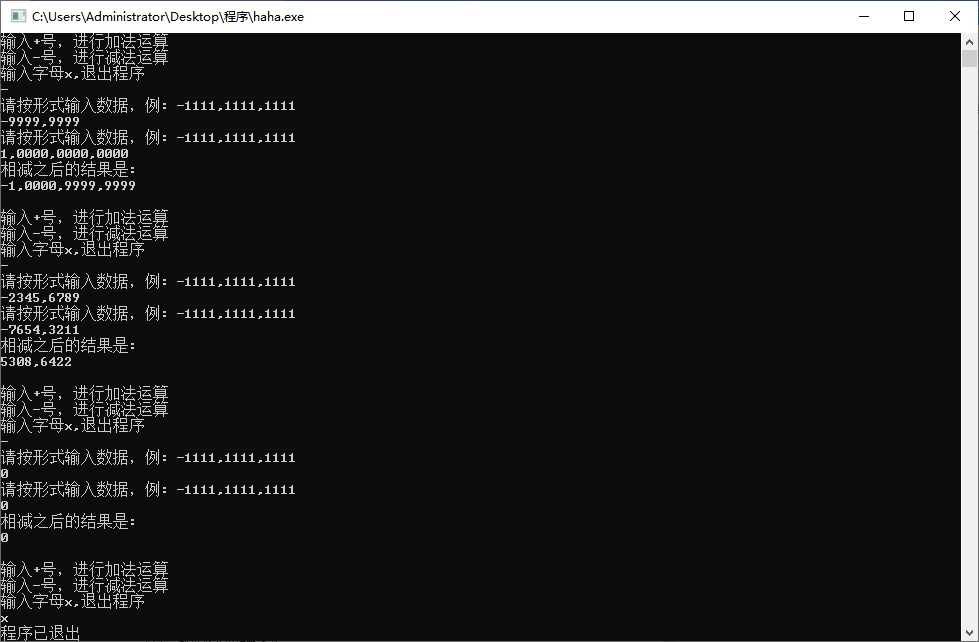• /* 函数circle用于判断整数n的d进制数表示形式是否是回文数 */ #include<stdio.h> #include<stdlib.h> int circle(int n, int d){ int s=0,m=n; while(m) { s=s*d+m%d; m/=d; } printf("s=%...
/* 函数circle用于判断正整数n的d进制数表示形式是否是回文数 */
#include<stdio.h>
#include<stdlib.h>

int circle(int n, int d){
int s=0,m=n;
while(m)
{
s=s*d+m%d;
m/=d;
}
printf("s=%d\n",s);
return s==n;
}
/* main函数用于测试circle函数 */
int num[]={232,27,851};
int scale[]={2,10,16};
main(){
int i,j;
system("cls");// clrscr();
for(i=0;i<sizeof(num)/sizeof(num);i++)
for(j=0;j<sizeof(scale)/sizeof(scale);j++)
if(circle(num[i],scale[j]))
printf("%d -> (%d) is a Circle Number!\n",num[i],scale[j]);
else
printf("%d -> (%d) is not a Circle Number!\n",num[i],scale[j]);
printf("\n Press any key to quit...\n");
getch();
}

其中以下代码为精华所在:

while(m)
{
s=s*d+m%d;
m/=d;
}
return s==n;

主要思想为把n倒序表示成s，最后判断s与n是否相等，相等即为回文数。

例如：判断数n=123,该进制下d=10是否为回文数？
step1: s=0*10+123%10=3;
m=123/10=12;
step2: s=3*10+12%10=32;
m=12/10=1;
step3: s=32*10+1%10=321;
m=1/10=0;
step4: m=0,退出while循环
step5: return s==n;(321==123判断为false,即该数不为回文数)

展开全文• C语言基础篇接下来给大家介绍一个用基础算法判断一个任意整数的位数并且正向逆向输出各位数。#include &lt;stdio.h&gt; #include &lt;math.h&gt; int main() { int a,b,c,d,e,f,g,h; int ...
C语言基础篇接下来给大家介绍一个用基础算法判断一个任意整数的位数并且正向逆向输出各位数。#include <stdio.h>
#include <math.h>

int main()
{
int a,b,c,d,e,f,g,h;
int count1 = 1,count2=1;
scanf("%d",&a);    //读取整数
c = a;
d=a;
f=a;
while(f / 10 != 0)   //用取余法判断位数
{
count1++;
g = f % 10;
f = f / 10;
}
printf("%d\n",count1);  //输出位数

while(a % 10 != 0)   //用整除法正向输出各个位数
{
h = a / (int)pow(10,(count1-1));
printf("%d ",h);
a = a % (int)pow(10,count1-1);
count1--;
}
printf("\n");
while(d / 10 != 0)  //用循环除10取余法逆向输出各个位数
{
e = d % 10;
printf("%d ",e);
d = d / 10;
}
e = d % 10;
printf("%d\n",e);
return 0;
} 希望有更好算法的网友可以在下方评论！第一次写，希望可以帮助到你们！
展开全文• ## C语言合法整数

千次阅读 2015-10-18 13:45:45
其实，刷题后，自己判断答案，还是上机自己实验一下，再得出结论，比较靠谱. // testvc6.cpp : Defines the entry point for the console application. // #include "stdafx.h" void fnTest(); int
刷题时，错了一道. 后来看了一下，想了一下，对于刷题时的我，再给我一次机会，这题也是必错的~
其实，刷题后，自己判断答案，还是上机自己实验一下，再得出结论，比较靠谱.
// testvc6.cpp : Defines the entry point for the console application.
//

#include "stdafx.h"

void fnTest();

int main(int argc, char* argv[])
{
fnTest();
getchar();
return 0;
}

void fnTest()
{
/**
以下选项中可以作为C语言合法整数的是(C)
A) 10110B
B) 0386
C) 0Xffa
D) x2a2
*/

int iTemp = 0;

/// C语言中无法直接表示二进制数, 需要用16进制数来表示2进制数
// iTemp = 10110B; ///< error C2059: syntax error : 'bad suffix on number'
iTemp = 0x16;

/// 以0开头的数表示的是8进制数, 而8进制数中是没有8的, 因为将要出现8就进位了
// iTemp = 0386; ///< error C2041: illegal digit '8' for base '8'
iTemp = 386;

iTemp = 0xffa; ///< 在工程中经常用0xXX表示16进制数
iTemp = 0Xffa; ///< 但是0X也表示16进制数

/// x2a2 赋值到一个整数，明显是错的.没有x这个数, 只有0x表示16进制数
// iTemp = x2a2; ///< error C2065: 'x2a2' : undeclared identifier
iTemp = 0x2a2;
}

展开全文• 设计算法，实现一个任意长的整数进行加法、减法运算的演示程序。例如：1234，5123，4512，3451，2345与-1111，1111，1111，1111，1111的加法结果为：0123，4012，3401，2340，1234。基本要求如下： （1） 利用链表...
**
题目如下
**
设计算法，实现一个任意长的整数进行加法、减法运算的演示程序。例如：1234，5123，4512，3451，2345与-1111，1111，1111，1111，1111的加法结果为：0123，4012，3401，2340，1234。基本要求如下：
（1）	利用链表实现长整数的存储，每个节点含一个整型变量；
（2）	整型变量的范围：-（2^15 -1）~（2^15 -1）；
（3）	输入与输出形式每四位一组，组间用逗号分隔开。如：1986,8213,1935,2736,3299；
（4）	界面友好，每步给出适当的操作提示，并且系统具有一定的容错能力。
至少给出下面的测试数据：
（1）0； 0
（2）-2345,6789；  -7654,3211
（3）-9999,9999；  1,0000,0000,0000
（4）1,0001,0001；  -1,0001,0001
（5）1,0001,0001；  -1,0001,0000
（6）-9999,9999,9999；  -9999,9999,9999
（7）1,0000,9999,9999；  1
**
C++代码如下
**
#include <stdio.h>
#include <stdlib.h>
#include <conio.h>
#include <ctype.h>

typedef struct DualNode
{
int data;
struct DualNode *prior, *next;
}DualNode, *DualList;

DualList InitList(int sign)
{
//头结点存放符号位，1为正，-1为负
DualList L;
L = (DualList)malloc(sizeof(DualNode));
L->next = L->prior = L;
L->data = sign;
return L;
}

void InsertNodeAtTail(DualList L, int data)
{
//尾插，用于存储数据的输入
DualNode *s;
s = (DualList)malloc(sizeof(DualNode));
s->data = data;
s->next = L;
s->prior = L->prior;
L->prior->next = s;
L->prior = s;
}

{
// 即插在头结点之后，用于计算结果的存储
DualNode *s;
s = (DualList)malloc(sizeof(DualNode));
s->data = data;
s->next = L->next;
s->prior = L;
L->next->prior = s;
L->next = s;
}

void PrintList(DualList L)
{
//打印结果
int FirstTime = 1;
DualNode *p = L;
if (p->data == -1) printf("-");
p = p->next;
while(p != L)
{
if (FirstTime)
{
FirstTime = 0;
printf("%d", p->data);
}
else
{
printf(",%04d", p->data);
}
p = p->next;
}
printf("\n");
}

DualList InputData()
{
int FirstNum = 1, data;
char c;
DualList L;
L = (DualList)malloc(sizeof(DualNode));
L->next = L->prior = L;
printf("请按形式输入数据，例: -1111,1111,1111\n");
fflush(stdin);//清除键盘缓冲区
if ((c = getchar()) == '-')
L = InitList(-1);
else
L = InitList(1);
if (isdigit(c))
// 退格处理
ungetc(c, stdin);
do{
scanf("%d", &data);
InsertNodeAtTail(L, data);
}while((c = getchar()) != '\n');
//printf("输入的数据是:\n");
//PrintList(L);
return L;
}

void DelNode(DualList L, DualNode *p)
{
p->prior->next = p->next;
p->next->prior = p->prior;
free(p);
}

void Add(DualList a, DualList b, DualList c)
{
DualList pa, pb;
int carry = 0, tmp;
pa = a->prior;
pb = b->prior;
while((pa!= a) && (pb!= b))
{
tmp = pa->data + pb->data + carry;
if (tmp >= 10000)
{
carry = 1;
tmp -= 10000;
}
else
carry = 0;
pa = pa->prior;
pb = pb->prior;
}
while(pa!= a)
{
// pb = b
tmp = pa->data + carry;
if (tmp >= 10000)
{
carry = 1;
tmp -= 10000;
}
else
carry = 0;
pa = pa->prior;
}
while(pb!= b)
{
// pa = a
tmp = pb->data + carry;
if (tmp >= 10000)
{
carry = 1;
tmp -= 10000;
}
else
carry = 0;
pb = pb->prior;
}
if (carry != 0)
}

void Sub(DualList a, DualList b, DualList c)
{
DualList pa, pb, pc;
int borrow = 0,tmp;
pa = a->prior;
pb = b->prior;
while((pa != a) && (pb != b))
{
if (pa->data >= pb->data + borrow)
{
tmp = pa->data - pb->data - borrow;
borrow = 0;;
}
else
{
tmp = 10000 + pa->data - pb->data - borrow;
borrow = 1;
}
pa = pa->prior;
pb = pb->prior;
}
if (pa != a || (pa == a && pb == b && borrow == 0))
{
// a >= b
c->data = a->data;
}
if (c->data != a->data)
{
// a < b
pc = c->prior;
while(pc != c)
{
// 结果转换
if (pc == c->prior)
pc->data = 10000 - pc->data;
else
pc->data = 9999 - pc->data;
pc = pc->prior;
}
// 因为符号判断错误，所以borrow要取反
borrow = borrow?0:1;
while(pb != b)
{
if (pb->data >= borrow)
{
tmp = pb->data - borrow;
borrow = 0;
}
// 继续借位
else
{
tmp = 10000 + pb->data - borrow;
borrow = 1;
}
pb = pb -> prior;
}
}
else{
// a>b
while(pa != a)
{
if (pa->data >= borrow)
{
tmp = pa->data - borrow;
borrow = 0;
}
else
{
tmp = 10000 - pa->data - borrow;
borrow = 1;
}
pa = pa->prior;
}
}
pc = c->next;
while(pc->next !=c && pc->data == 0)
{
pc = pc->next;
DelNode(c, pc->prior);
}
}

{
DualList c;
if (a->data * b->data > 0)
{
c = InitList(a->data);
}else
{
c=InitList(b->data);
Sub(a, b, c);
}
if(c->next->data==0)
c->data=1;
return c;
}
DualList SubList(DualList a, DualList b)
{
DualList c;
if (a->data * b->data > 0)
{
if((a->data==-1)&&(b->data==-1))
{
b->data=1;
c=InitList(b->data);
Sub(a, b, c);
}
else
{
b->data=1;
c=InitList(b->data);
Sub(a,b,c);
}
}
else
{
c=InitList(b->data);
if((a->data==1)&&(b->data==-1))
c->data=1;
else c->data=-1;
}
if(c->next->data==0)
c->data=1;
return c;
}

int main()
{
char sign;
DualList a, b, c;
do{
printf("输入+号，进行加法运算\n");
printf("输入-号，进行减法运算\n");
printf("输入字母x,退出程序\n");
scanf("%s",&sign);
switch(sign)
{
case'+':
a = InputData();
b = InputData();
printf("相加之后的结果是:\n");
PrintList(c);
printf("\n");
break;
case'-':
a = InputData();
b = InputData();
c = SubList(a, b);
printf("相减之后的结果是:\n");
PrintList(c);
printf("\n");
break;
case'x':
break;
case'X':
break;
default:
printf("选择错误，请重新选择\n");
}
}while(sign!='x'&&sign!='X');
printf("程序已退出\n");
return 0;
}


**
程序运行结果截图如下
**展开全文算法 编程语言
• C语言 输出整数的逆序数 注意情况： 整数为负数； 整数的尾数为0。 #include<stdio.h> #include<math.h> int change(int n); int main() { int n; scanf("%d",&n); change(n);//运行函数将n...
• 在C中来判断输入的是浮点数是整数的DEMO。通过表达式m-(int)m是否大于0来判断或者把输入作为字符串读入，然后检索字符串中是否有"."来判断#include #define FIRST_DEMO //计算浮点数的位数//#define SECOND_DEMO//#...
• #include &lt;stdio.h&gt; #define is_power_2(x) ((x&gt;0) &amp;&amp; (0 == (x&amp;(x-1)))) int main() { for(int i=0; i&lt;63; i++) { printf("...
• 用户输入三个整数判断这三个整数能否构成三角形 #include <stdio.h> int main(void) { int a,b,c; printf("请输入3个整数\n"); scanf("%d" "%d" "%d",&a,&b,&c); if (a+b>c && ...算法
• /*本题要求实现一个计算非负整数阶乘的简单函数 其中N是用户传入的参数，其值不超过12。 如果N是非负整数，则该函数必须返回N的阶乘，否则返回0。 */ /* *非负整数的阶乘 *2019-9-29 *qq_2967953656 *version ...
• 文章目录【C】C语言判断字符串是否是int型正整数前言一、代码二、结果 【C】C语言判断字符串是否是int型正整数 前言 &nbsp;&nbsp;&nbsp;&nbsp;&nbsp;&nbsp;&nbsp;&nbsp;此程序功能...
• 在 C 语言中，除了 0 为 false，其余整数都为 true。
• 编写一个C程序，输入a,b,c的值，输出其中最大者 实现过程 1、比较从第一个数开始，先比较第一个数和第二个数的大小，如果第一个数大于第二个数，则将第一个数和第二个数互换；不大于（即等于或小于）则不作处理。...
• ## C语言判断一个数是否为整数

万次阅读 多人点赞 2018-12-29 10:45:13
为了准备考研，已是近半年未曾写过博客，...C语言判断一个数是否为整数，这是一个很常见但是又经常困扰我的一件事，其实也很简单。 对于输入的double a;使用floor(a+0.5) == a来判断即可。 原因如下：floor()是向...算法
• C语言实验-7.4-编写程序。判断整数m是否是素数
• C语言 统计整数二进制表示中1的个数 这是一个很有意思的问题，也是在面试中最容易被问到的问题之一。这个问题有个正式的名字叫Hamming_weight，而且wikipedia上也提供了很好的位运算解决的方法，这个下面也...二进制
• 整数的位数及各位数字之和 题目描述： 对于给定的正整数N，求它的位数及其各位数字之和。 输入格式： 输入在一行中给出一个不超过10 ​9 ​​ 的正整数N。 输出格式： 在一行中输出N的位数及其各位数字之和，中间...
• 计蒜客——整数转化为罗马数字的一点体会： 首先想到的思路是单独一个一个的把输入的数字拆分开来，每一位对应的数字转化成罗马数字，建立{由于题目限制}（1-3999）四个数组分别代表unit、ten、hundred、thousands...
• 整数的逆序输出 1.算法思路 利用%取余运算，根据不同进制数数据采用不同； 对取得余数进行逆运算（乘以刚才除数），然后加上刚才取得余数； 循环上一步； 2、代码如下： // 整数的逆序输出 #include <stdio.h&...
• 题目： j将一个 32 位的有符号整数，要将这个整数中每一位数字进行反转。 假设我们的环境只能存储得下 32 位的有符号整数，第1位为符号位和后32位，其数值范围为 ...超过这个范围就溢出，所以我们要判断，如果前8位相等leetcode
• 对于给定的正整数 N，求它的位数及其各位数字之和。 输入格式： 输入在一行中给出一个不超过 10 ​9 ​​ 的正整数 N。 输出格式： 在一行中输出 N 的位数及其各位数字之和，中间用一个空格隔开。 我的答案 #include...
• 一、问题重述 【问题描述】 从标准输入中读入一个整数算术运算表达式，如5 - 1 * 2 * 3 + 12 / 2 / 2 = 。计算表达式结果，并输出。...3、出现除号/时，以整数相除进行运算，结果仍为整数，例如：5/3结果应
• C语言判断一个double型数是否为数学上的整数 例：判断整数x的平方根是否为整数(即判断x是否为完全平方数) #include <stdio.h> #include <math.h> int main () { int x; double m,n,k; scanf("%d",&x...基础
• 判断输入的正整数是否是5和7的整数倍。若是，输出yes，否则输出No。 用if来判断 #include &amp;amp;lt;stdio.h&amp;amp;gt; void main() { int x;/*定义输入的数为整数*/ printf(&amp;quot;input x=\n...if
• 判断一个整数的位数， 平常我们这样判断一个整数简答代码是这样的 int Bit_Int(long n) { int bit=0; if(n==0) return 1; while(n) { bit++; n/=10; } return bit; } 现在我学到了这个这一行代码 ...
• ## C语言判断素数

千次阅读 多人点赞 2019-05-15 22:48:16
素数又称质数。...思路1：因此判断一个整数m是否是素数，只需把 m 被 2 ~ m-1 之间的每一个整数去除，如果都不能被整除，那么 m 就是一个素数。 思路2：判断方法还可以简化。m 不必被 2 ~ m-1 之间...
• 整数在内存中的存储 整型数就是通常使用的整数，分为无符号整数和带符号整数两大类。内存 二进制 存储
• BigInt大整数运算库 前言 这个版本为第一个版本，未有太多优化。大多采用类似手算的算法 这个算法内存占用过大。自定义的结构体数字表示采用定长的方法，后期将优化采用动态长度的方法，减少内存占用。 B......

# c语言判断整数c语言 订阅